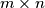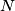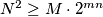### IMO Shortlist 2005 problem C3

Kvaliteta:
Avg: 0.0
Težina:
Avg: 7.0
Consider a$m\times n$ rectangular board consisting of$mn$ unit squares. Two of its unit squares are called adjacent if they have a common edge, and a path is a sequence of unit squares in which any two consecutive squares are adjacent. Two parths are called non-intersecting if they don't share any common squares.

Each unit square of the rectangular board can be colored black or white. We speak of a coloring of the board if all its$mn$ unit squares are colored.

Let$N$ be the number of colorings of the board such that there exists at least one black path from the left edge of the board to its right edge. Let$M$ be the number of colorings of the board for which there exist at least two non-intersecting black paths from the left edge of the board to its right edge.

Prove that$N^{2}\geq M\cdot 2^{mn}$.
Source: Međunarodna matematička olimpijada, shortlist 2005